# The Rational Class

Like other numbers, we think of a rational number as a unit, but all rational numbers can be most easily represented as a fraction, a ratio of two integers. We can create a class Rational, so

```Rational r = new Rational(2, 3);
```

would create a new Rational number for the mathematical expression, 2/3.

Our previous simple class examples have mostly been ways of collecting related data, with limited methods beyond getters and setters. Rational numbers also have lots of obvious operations defined on them. Our Rational example we will use most of the concepts for object so far, and add a few more, to provide a rich practical class with a variety of methods.

Thinking ahead to what we would like for our rational numbers, here is some testing code. Hopefully the method names are clear and reasonable. in the illustration we operate on a single rational number, and do calculation with a pair, and parse string literals. The code is from test_rational/test_rational.cs:

```using System;

namespace IntroCS
{
public class TestRational
{
public static void Main()
{
Rational f = new Rational(6, -10);
Console.WriteLine("6/(-10) simplifies to {0}", f);
Console.WriteLine("reciprocal of {0} is {1}", f, f.Reciprocal());
Console.WriteLine("{0} negated is {1}", f, f.Negate());
Rational h = new Rational(1, 2);
Console.WriteLine("{0} + {1} is {2}", f, h, f.Add(h));
Console.WriteLine("{0} - {1} is {2}", f, h, f.Subtract(h));
Console.WriteLine("{0} * {1} is {2}", f, h, f.Multiply(h));
Console.WriteLine("({0}) / ({1}) is {2}", f, h, f.Divide(h));
Console.WriteLine("{0} > {1} ? {2}", h, f, (h.CompareTo(f) > 0));
Console.WriteLine("{0} as a double is {1}", f, f.ToDouble());
Console.WriteLine("{0} as a decimal is {1}", h, h.ToDecimal());
foreach (string s in new[] {"-12/30", "123", "1.125"}) {
Console.WriteLine("Parse \"{0}\" to Rational: {1}",
s, Rational.Parse(s));
}
}
}
}
```

One non-obvious method is `CompareTo`. This one method allows all the usual comparison operators to be used with the result. We will discuss it more in Rationals Revisited.

The results we would like when running this testing code:

```6/(-10) simplifies to -3/5
reciprocal of -3/5 is -5/3
-3/5 negated is 3/5
-3/5 + 1/2 is -1/10
-3/5 - 1/2 is -11/10
-3/5 * 1/2 is -3/10
(-3/5) / (1/2) is -6/5
1/2 > -3/5 ? true
-3/5 as a double is -0.6
1/2 as a decimal is 0.5
Parse "-12/30" to Rational: -2/5
Parse "123" to Rational: 123
Parse "1.125" to Rational: 9/8
```

A Rational has a numerator and a denominator. We must remember that data. Each individual Rational that we use will have its own numerator and denominator, which we store as the instance variables (and which we abbreviate since we are lazy):

```public class Rational
{
private int num;
private int denom;
// ...
```

We could have a very simple constructor that just copies in values for the numerator and denominator. However, there is an extra wrinkle with rational numbers: They can be represented many ways. You remember from grade school being told to “reduce to lowest terms”. This will keep our internal representations unique, and use the smallest numbers.

Intermediate operations and initial constructor parameters will not always be in lowest terms. To reduce to lowest terms we need to divide the original numerator and denominator by their Greatest Common Divisor. We include a static GCD method taken from that section, and make the adjustment to lowest terms in a helping method, `Normalize`, that is called by the constructor:

```      /// Force the invarient: in lowest terms with a positive denominator.
private void Normalize()
{
if (denom == 0) { // We *should* force an Exception, but we won't.
Console.WriteLine("Zero denominator changed to 1!");
denom = 1;
}
int n = GCD(num, denom);
num /= n;         // lowest
denom /= n;       //    terms
if (denom < 0) {  // make denom positive
denom = -denom;//   double negation:
num = -num;    //   same mathematical value
}
}
```

• It is private. It is only used as a helping method, called from inside of Rational. It is not a part of the public interface used from other classes.

• We need to deal with a 0 denominator somehow. We should be causing an exception, but that is an advanced topic, so we wimp out and just change the denominator to 1.

• There is one other technical issue in getting a unique representation: The denominator could start off being negative. If that is the case, we change the sign of both the numerator and denominator, so we always end up with a positive denominator. We will use this fact in several places.

• It calls a static method of the class, `GCD`. Classes can have both instance and static methods. It is fine for an instance method like `Normalize` to call a static method: The instance variables cannot be accessed. Here `GCD` is passed all its data explicitly through its parameters.

The complete constructor, using `Normalize`, is below. Note that by the time we are done constructing a new Rational, it is in this normalized form: lowest terms and positive denominator:

```      /// Create a fraction given numerator and denominator.
public Rational(int numerator, int denominator)
{
num = numerator;
denom = denominator;
Normalize();
}
```

The call to the `Normalize` method is another place where we have a call without dot notation, since it is acting on the same object as for the constructor.

We mentioned that instance method `Normalize` calls static method `GCD`, and this is fine. The reverse is not true:

Warning

Inside a `static` method there is no current object. A common compiler error is caused when you try to have a static method call an instance method without dot notation for a specific object. The shorthand notation without an explicit object reference and dot cannot be used, because there is no current object to reference implicitly:

```public void AnInstanceMethod()
{
...
}

public static void AStaticMethod()  // no current object
{
AnInstanceMethod();  // COMPILER ERROR CAUSED
}
```

On the other hand, there is no issue when an instance method calls a static method. (The instance variables are just inaccessible inside the static method.)

The Rational class has the usual getter methods, to access the obvious parts of its state:

```      public int GetNumerator()
{
return num;
}

public int GetDenominator()
{
return denom;
}
```

We certainly want to be able to display a Rational as a string version:

```      /// Return a string of the fraction in lowest terms,
/// omitting the denominator if it is 1.
public override string ToString()
{
if (denom != 1)
return string.Format("{0}/{1}", num, denom);
return ""+num;
}
```

Note that we simplify so you would see “3” rather than “3/1”. This is also a place where the normalization to have a positive denominator comes in: a negative Rational will always have a leading “-” as in “-5/9” rather than “5/-9”

With a Rational, several other conversions make sense: to `double` and `decimal` approximations.

```      /// Return a double approximation to the fraction.
public double ToDouble()
{
return ((double)num)/denom;
}

/// Return a decimal approximation to the fraction.
public decimal ToDecimal()
{
return ((decimal)num)/denom;
}
```

So far we have returned built-in types. What if we wanted to generate the reciprocal of a Rational? That would be another Rational. It is legal to return the type of the class that you are defining! How do we make a new Rational? We have a constructor! We can easily use it. The reciprocal swaps the numerator and denominator. It is also easy to negate a Rational:

```      /// Return a new Rational which is the reciprocal of this Rational.
public Rational Reciprocal()
{
return new Rational(denom, num);
}

/// Return a new Rational which is this Rational negated.
public Rational Negate()
{
return new Rational(-num, denom);
}
```

Static methods are still useful. For example, in analogy with the other numeric types we may want a static `Parse` method to act on a string parameter and return a new Rational.

The most obvious kind of string to parse would be one like `"2/3"` or `"-10/77"`, which we can split at the `'/'`. Integers are also rational numbers, so we would like to parse `"123"`. Finally decimal strings can be converted to rational numbers, so we would like to parse `"123.45"`.

See how our `Parse` method below distinguishes and handles all the cases. It constructs integer strings, `parts` and `parts`, for both the numerator and denominator, and then parses the integers. Note that the method is `static`. There is no Rational being referred to when it starts, but in this case the method returns one.

That last case is the trickiest. For example `"123.45"` becomes 12345/100 (before being reduced to lowest terms). Note that there were originally two digits after the decimal point and then the denominator gets two zeroes to have the right power of 10:

```      /// Parse a string of an integer, fraction (with /) or decimal (with .)
///  and return the corresponding Rational.
public static Rational Parse(string s)
{
s = s.Trim();      // will adjust numerator and denominator parts
string[] parts = {s, "1"}; // for an int string, this is correct
if (s.Contains("/")) {     // otherwise correct num, denom parts
parts = s.Split('/');
} else if (s.Contains(".")) {
string[] intFrac = s.Split('.'); // integer, fractional digits
parts = intFrac+intFrac;  // "shift" decimal point
parts = "1";                 // denom will have as many 0's
foreach( char dig in intFrac) {  //    as digits after '.'
parts += "0";                 //    to compensate
}
}
return new Rational(int.Parse(parts.Trim()),
int.Parse(parts.Trim()));
}
```

## Method Parameters of the Same Type

We can deal with the current object without using dot notation. What if we are dealing with more than one Rational, the current one and another one, like the parameter in Multiply:

```      /// Return a new Rational which is the product of this Rational and f.
public Rational Multiply(Rational f)
```

We can mix the shorthand notation for the current object’s fields and dot notation for another named object: `num` and `denom` refer to the fields in the current object, and `f.num` and `f.denom` refer to fields for the other `Rational`, the parameter `f`.

```      /// Return a new Rational which is the product of this Rational and f.
public Rational Multiply(Rational f)
{  // end Multiply heading chunk
return new Rational(num*f.num, denom*f.denom);
}
```

We do not refer to the fields of `f` through the public methods `GetNumerator` and `GetDenominator`. Though `f` is not the same object, it is the same type:

Note

Private members of another object of the same type are accessible from method definitions in the class.

There are a number of other arithmetic methods in the source code for Rational that return a new Rational result of the arithmetic operation. They do review your knowledge of arithmetic! They do not add further C# syntax.

The whole code for Rational is in rational_nunit/rational.cs. The testing code we started with, in test_rational/test_rational.cs uses all the methods. We will see more advance ways to test Rational in Testing.

There is also a more convenient version of Rational, using advanced concepts, in Defining Operators (Optional).

## Pictorial Playing Computer

Let us start pictorially playing computer on `test_rational.cs`, as a review of much of the previous sections. We explicitly show a local variable `this` to identify the current object in an instance method or constructor.

The first line of `Main`,

```Rational f = new Rational(6, -10);
```

creates a new Rational, so it calls the constructor. At the very beginning of the constructor, a prototype `Rational` is already created as the current object, so immediately, there is a `this`. The parameters 6, and -10 are passed, initializing the explicit local variables `numerator` and `denominator`. The figure illustrates the memory state at the beginning of the constructor call:Note the immediate value assigned to the numeric instance variables is zero: This is as discussed in Default Initializations. Of course we do not want to keep those default values: The constructor finds the value of the local variable `numerator`, and needs to assign the value 6 to a variable `num`. The compiler has looked first for a local variable `num`, and found none. Then it looked second for an instance variable in the object pointed to by `this`. It found `num` there. Now it copies the 6 into that location. Similarly for `denominator` and `denom`: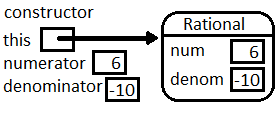Then the constructor calls `Normalize`. Since `Normalize` is also an instance method, a reference to `this` is passed implicitly. While illustrating the memory state for more than one active method, we separate each one with a horizontal segment.Later `Normalize` calls `GCD`. Since `GCD` is static, note that the local variables for `GCD` do not contain a reference to `this`.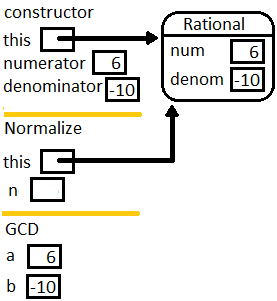At the end of `GCD` the `int` 2 is returned and initializes `n` in the calling method `Normalize`. Then `Normalize` modifies the instance variable pointed to by `this`, and finishes.That is the same object `this` in the constructor. Just before the constructor completes we have:Then in `Main` the constructor’s `this` is the reference to the new object initializing `f`.Consider the next line of `Main`:

```Console.WriteLine("6/(-10) simplifies to {0}", f);
```

We omit the internals of the WriteLine call, except to note that it must convert the reference `f` to a string. As with any object, it does this by calling the `ToString` method for `f`, so the implicit `this` in the call to `ToString` refers to the same object as `f`: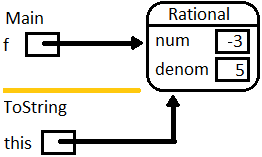`ToString` returns “-3/5”, and it gets printed as part of the line generated by `WriteLine`….

We skip the similar details through two more `WriteLine` statements and the initialization of `h`:

```Rational h = new Rational(1,2);
```

The `WriteLine` statement after that needs to evaluate `f.Add(h)`, generating a call to `Add`. The next figure shows the two local variables in `Main`, `f` and `h`, each pointing to a `Rational` object. The image shows the situation in the call to `Add`, just before the end of the return statement, when the new Rational is being constructed.

In the local variables for the method `Add` see what the implicit `this` refers to, and what the (local to `Add`) variable `f` refer to. As the figure shows, this use of a local variable `f` is independent of the `f` in `Main`:Since the return statement in `Add` creates a new object, the figure shows a call to the constructor from inside `Add`. We do not go through the details of another constructor call, but `this` in the constructor points to the Rational shown and returned by `Add`: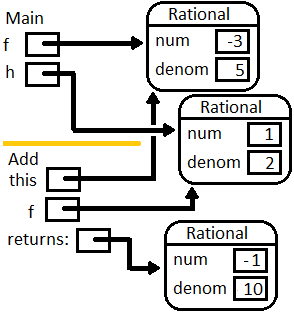which gets sent to the `WriteLine` statement and gets printed in `Main` as in the earlier code.

Make sure you see how the pictures reinforce these important ideas:

• Keeping track of the `this` with constructors and instance methods (but not static methods).

• The aliasing of `Rational` objects used as parameters explicitly or implicitly (`this`).

We have played computer before in procedural programming, following individually explicitly named variables. This has allowed us to follow loops clearly after the code is written. The pictorial version with multiple object references and method calls is also useful for checking on code that is written with many object references.

When first writing code with object references that you are manipulating, a picture of the setup showing the references in your data is also helpful. New object-oriented programmers often have a hard time referring to the data they want to work with. If you have a picture of the data relationships you can point with a finger to a part that you want to use. For example in the call to `Add`, one piece of data you need for your arithmetic is the `num` field in `f`. Then you must be carful to note that only local variables can be referenced directly (including the implicit `this`). If you want to refer to data that is not a local variable, you must follow the reference path arrow that leads from a local variable to an instance field that you want to reference.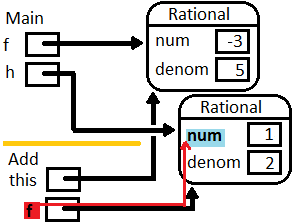Then use the proper object-oriented notation to refer to the path. In the example, it takes one step, from local variable `f` to its field `num`, referred to as `f.num`. Similarly the current object’s `num` is connected through `this`, but C# shorthand allows `this.` to be omitted. And so on, for `f.denom` and `denom`.

Visually following such paths will be even more important later, when we construct more complex types of objects, and you need to follow a path through several references in sequence.

## ForceMatch Exercise

Suppose we have a class:

```class Pair
{
private int x,y;

public Pair(int x, int y)
{
this.x = x; this.y = y;
}

///Mutate the parameter so its instance variables match this object
public void ForceMatch(Pair p)
{
// need code ...
}

public override string ToString()
{
return string.Format("({0}, {1})", x, y);
}
}
```

A test would be code in another class:

```Pair first = new Pair(3, 7);
Pair second = new Pair(1, 9)
Console.WriteLine(second);  // prints (1, 9)
first.ForceMatch(second);
Console.WriteLine(second);  // prints (3, 7)
```
1. Would this code work? If not, explain why not:

```public void ForceMatch(Pair p)
{
p = new Pair(x, y);
}
```

If you do not see it, do a graphical play-computer like in the last section.

2. Complete the body of `ForceMatch` correctly.